# Overview

“The metapopulation concept is to subdivide the entire population into distinct ‘subpopulations’, each of which has independent dynamics, together with limited interaction between the subpopulations.” - Keeling MJ and Rohani Pejman (Modeling Infectious Diseases In Humans and Animals).

In this project, I aim to use metapopulation dynamics to model malaria transmission between heterogenous subpopulations while accounting for varying definitions and degrees of intermixing.

# Model

## Model Overview

These are modified SEIR/SIR compartmental models accounting for two species across three subpopulations .

### Compartments

My first model will consist of four distinct compartments for each host subpopulation and three distinct compartments for each vector subpopulation reflecting the natural history/biology of malaria. For example, in population A, there will be:

• $$S_{A,host}$$ - uninfected, susceptible hosts
• $$E_{A,host}$$ - exposed, non-infectious hosts
• $$I_{A,host}$$ - infected, infectious hosts
• $$R_{A,host}$$ - recovered, removed hosts

• $$S_{A,vector}$$ - susceptible vectors
• $$E_{A,vector}$$ - exposed, non-infectious vectors
• $$I_{A,vector}$$ - infected, infectious vectors

We assume that once a vector is infected, it stays infected until it dies. Therefore, recovered vectors are not included in the model.

The second model is a simplified version of the one aforementioned. The only differences are the exclusion of the Exposed compartments for both hosts and vectors.

### Processes

The processes being modeled within each subpopulation are:

• Susceptible hosts can become exposed by contact with infectious vectors at rate $$b_1$$
• Susceptible vectors can become exposed by contact with infectious hosts at rate $$b_2$$

• Exposed hosts become infected/infectious after some time reflecting Plasmodium spp. life cycle, infection–>hepatic stage–>erythrocytic stage (specified by the rate $$incu_{*,host}$$) ~ 9-14 days

• Exposed vectors become infected/infectious after some time reflecting Plasmodium spp. life cycle, maturation (specified by the rate $$incu_{*,vector}$$) ~ 10-18 days

• Infected hosts recover after some time (specified by the rate $$g$$). ~ 2 weeks average (vary within populations)

• New susceptible vectors are born at a rate $$br$$

• Susceptible and infected vectors die at rate $$dr$$
• The inverse of this rate is the average lifespan.

• Recovered hosts lose their immunity at rate $$w$$.

Additionally, I introduce metapopulation parameters to account for movement between . I elaborate further in a following section.

#### Population Intermixing

The interaction between somewhat distinct subpopulations can be characterized in a variety of different ways. Common approaches to the parameterizing population intermixing have consisted of random intermixing between subpopulations and also introduced the “visitor” concept. For the random intermixing between subpopulations, there are two common assumptions made: (1) net migration is zero allowing for stable population sizes and (2) there exists equality in migration direction, e.g., migration from population A to population B equals migration from population B to population A. For the “visitor” concept, human population movement has been observed to be largely not permanent. This leads us to consider both native and foreign populations differently when modeling our metapopulation dynamics.

## Simulation Functions

### Random intermixing between subpopulations

Principally of note in the below code, there are nine coupling parameters. They are prefixed by “m” and have two letters following which designate from and to populations; e.g., m.AB represents the coefficient for movement from population A to population B. Initial settings for the simulation set m.AA=m.BB=m.CC=1, representing zero migration and three distinct models. The sum of the migration parameters for any one destination should be one, so that they represent the proportion of populations migrating. The total sum of all migration parameters should be three(?) so that the total population remains stable.

So, I outline processes defining movement:

• $$m_{AB}$$ - migration from A to B,
• $$m_{AC}$$ - migration from A to C,
• $$m_{BA}$$ - migration from B to A,
• $$m_{BC}$$ - migration from B to C,
• $$m_{CA}$$ - migration from C to A,
• $$m_{CB}$$ - migration from C to B.
METAPOP.eq <- function(t, y, parms)
{
with(
as.list(c(y,parms)),
{
#the ordinary differential equations

dSh.A = -Sh.A*b1.A*Iv.A+m.AA*w.A*Rh.A-(m.AB+m.AC)*Sh.A+m.BA*Sh.B+m.CA*Sh.C+m.BA*w.B*Rh.B+m.CA*w.C*Rh.C
dEh.A = m.AA*Sh.A*b1.A*Iv.A-incu*Eh.A+m.BA*Sh.B*b1.B*Iv.B+m.CA*Sh.C*b1.C*Iv.C+m.BA*Eh.B+m.CA*Eh.C-(m.AB+m.AC)*Eh.A
dIh.A = m.AA*incu*Eh.A-g.A*Ih.A+m.BA*incu*Eh.B+m.CA*incu*Eh.C+m.BA*Ih.B+m.CA*Ih.C-(m.AB+m.AC)*Ih.A
dRh.A = m.AA*g.A*Ih.A-w.A*Rh.A+m.BA*g.B*Ih.B+m.CA*g.C*Ih.C-(m.AB+m.AC)*Rh.A+m.BA*Rh.B+m.CA*Rh.C

dSv.A = bir.A*(Sv.A+Ev.A+Iv.A)-dea.A*Sv.A-b2.A*Ih.A*Sv.A
dEv.A = -dea.A*Ev.A+b2.A*Ih.A*Sv.A-ma*Ev.A
dIv.A = -dea.A*Iv.A+ma*Ev.A

dSh.B = -Sh.B*b1.B*Iv.B+m.BB*w.B*Rh.B-(m.BA+m.BC)*Sh.B+m.AB*Sh.A+m.CB*Sh.C+m.AB*w.A*Rh.A+m.CB*w.C*Rh.C
dEh.B = m.BB*Sh.B*b1.B*Iv.B-incu*Eh.B+m.AB*Sh.A*b1.A*Iv.A+m.CB*Sh.C*b1.C*Iv.C+m.AB*Eh.A+m.CB*Eh.B-(m.BA+m.BC)*Eh.B
dIh.B = m.BB*incu*Eh.B-g.B*Ih.B+m.AB*incu*Eh.A+m.CB*incu*Eh.C+m.AB*Ih.A+m.CB*Ih.C-(m.BA+m.BC)*Ih.B
dRh.B = m.BB*g.B*Ih.B-w.B*Rh.B+m.AB*g.A*Ih.A+m.CB*g.C*Ih.C-(m.BA+m.BC)*Rh.B+m.AB*Rh.A+m.CB*Rh.C

dSv.B = bir.B*(Sv.B+Ev.B+Iv.B)-dea.B*Sv.B-b2.B*Ih.B*Sv.B
dEv.B = -dea.B*Ev.B+b2.B*Ih.B*Sv.B-ma*Ev.B
dIv.B = -dea.B*Iv.B+ma*Ev.B

dSh.C = -Sh.C*b1.C*Iv.C+m.CC*w.C*Rh.C-(m.CA+m.CB)*Sh.C+m.AC*Sh.A+m.BC*Sh.B+m.AC*w.A*Rh.A+m.BC*w.B*Rh.B
dEh.C = m.CC*Sh.C*b1.C*Iv.C-incu*Eh.C+m.BC*Sh.B*b1.B*Iv.B+m.AC*Sh.A*b1.A*Iv.A+m.BC*Eh.B+m.AC*Eh.A-(m.CA+m.CB)*Eh.C
dIh.C = m.CC*incu*Eh.C-g.C*Ih.C+m.BC*incu*Eh.B+m.AC*incu*Eh.A+m.AC*Ih.C+m.BC*Ih.B-(m.CA+m.CB)*Ih.C
dRh.C = m.CC*g.C*Ih.C-w.C*Rh.C+m.BC*g.B*Ih.B+m.AC*g.A*Ih.A-(m.CA+m.CB)*Rh.C+m.BC*Rh.B+m.AC*Rh.A

dSv.C = bir.C*(Sv.C+Ev.C+Iv.C)-dea.C*Sv.C-b2.C*Ih.C*Sv.C
dEv.C = -dea.C*Ev.C+b2.C*Ih.C*Sv.C-ma*Ev.C
dIv.C = -dea.C*Iv.C+ma*Ev.C

list(c(dSh.A, dEh.A, dIh.A, dRh.A, dSh.B, dEh.B, dIh.B, dRh.B, dSh.C, dEh.C, dIh.C, dRh.C, dSv.A , dEv.A , dIv.A , dSv.B , dEv.B , dIv.B , dSv.C , dEv.C , dIv.C ))
}
)
}

#' Simulation of a compartmental infectious disease transmission model illustrating metapopulation dynamics
#'
#' @description  This model allows for the simulation of a vector-borne infectious disease with metapopulation dynamics
#'
#'
#' @param Sh0.* initial number of susceptible hosts in population *
#' @param Eh0.* initial number of exposed hosts in population *
#' @param Ih0.* initial number of infectious hosts in population *
#' @param Sv0.* initial number of susceptible vectors in population *
#' @param Ev0.* initial number of exposed vectors in population *
#' @param Iv0.* initial number of infected vectors in population *
#'
#' @param tmax maximum simulation time, units of months
#' @param b1.* rate of transmission from infected vector to susceptible host in population *
#' @param b2.* rate of transmission from infected host to susceptible vector in population *
#' @param bir.* the rate of births of vectors in population *
#' @param dea.* the rate of death of vectors in population *
#' @param g.* the rate at which infected hosts recover in population *
#' @param w.* the rate at which host immunity wanes in population *
#' @param incu the rate at which the parasite enters a sexual reproductive phase
#' @param ma the rate at which the parasite matures to allow transmission to hosts
#'
#'
#' @return This function returns the simulation result as obtained from a call
#'   to the deSolve ode solver
#' @details A compartmental ID model with several states/compartments
#'   is simulated as a set of ordinary differential
#'   equations. The compartments are Sh.*, Eh.*, Ih.*, Rh.*, and Sv.*, Ev.*, Iv.* across * populations.
#'   The function returns the output from the odesolver as a matrix,
#'   with one column per compartment/variable. The first column is time.
#' @section Warning:
#'   This function does not perform any error checking. So if you try to do
#'   something nonsensical (e.g. any negative values or fractions > 1),
#'   the code will likely abort with an error message
#' @examples
#'   # To run the simulation with default parameters just call this function
#'   result <- simulate_metapopulation()
#'   # To choose parameter values other than the standard one, specify them e.g. like such
#'   result <- simulate_metapopulation(Sh0.A = 100, Sv0.A = 1e5,  tmax = 100)
#'   # You should then use the simulation result returned from the function, e.g. like this:
#'   plot(result[,1],result[,2],xlab='Time',ylab='Number Susceptible',type='l')
#' @seealso The UI of the shiny app 'Metapopulation', which is part of this package, contains more details on the model
#' @author Cody Dailey, Andreas Handel
#' @references See the information in the corresponding shiny app for model details
#'            See the documentation for the deSolve package for details on ODE solvers
#' @export

simulate_metapopulation <- function( Sh0.A = 1e2,      Sh0.B = 1e2,      Sh0.C = 1e2,
Eh0.A = 1,        Eh0.B = 0,        Eh0.C = 0,
Ih0.A = 0,        Ih0.B = 0,        Ih0.C = 0,
Rh0.A = 0,        Rh0.B = 0,        Rh0.C = 0,
Sv0.A = 1e2,      Sv0.B = 1e2,      Sv0.C = 1e2,
Ev0.A = 0,        Ev0.B = 0,        Ev0.C = 0,
Iv0.A = 0,        Iv0.B = 0,        Iv0.C = 0,

tmax = 1000,

b1.A = 0.01,      b1.B = 0.01,      b1.C = 0.01,
b2.A = 0.5,       b2.B = 0.5,       b2.C = 0.5,

bir.A = 1/25,     bir.B = 1/25,     bir.C = 1/25,
dea.A = 0.001,    dea.B = 0.001,    dea.C = 0.001,

g.A = 2,          g.B = 2,          g.C = 2,
w.A = 0.75,       w.B = 0.75,       w.C = 0.75,

incu = 3,
ma = 2,

m.AA = 1, m.AB = 0, m.AC = 0,
m.BB = 1, m.BA = 0, m.BC = 0,
m.CC = 1, m.CA = 0, m.CB = 0 )
{
############################################################

Y0 = c(Sh.A = Sh0.A, Eh.A = Eh0.A, Ih.A = Ih0.A, Rh.A = Rh0.A,
Sh.B = Sh0.B, Eh.B = Eh0.B, Ih.B = Ih0.B, Rh.B = Rh0.B,
Sh.C = Sh0.C, Eh.C = Eh0.C, Ih.C = Ih0.C, Rh.C = Rh0.C,
Sv.A = Sv0.A, Ev.A = Ev0.A, Iv.A = Iv0.A,
Sv.B = Sv0.B, Ev.B = Ev0.B, Iv.B = Iv0.B,
Sv.C = Sv0.C, Ev.C = Ev0.C, Iv.C = Iv0.C);  #combine initial conditions into a vector

dt = min(0.1, tmax / 1000); #time step for which to get results back

timevec = seq(0, tmax, dt); #vector of times for which solution is returned (not that internal timestep of the integrator is different)

############################################################
#vector of parameters which is sent to the ODE function
pars=c(b1.A = b1.A, b1.B = b1.B, b1.C = b1.C,
b2.A = b2.A, b2.B = b2.B, b2.C = b2.C,
bir.A = bir.A, bir.B = bir.B, bir.C = bir.C,
dea.A = dea.A, dea.B = dea.B, dea.C = dea.C,
g.A = g.A, g.B = g.B, g.C = g.C,
w.A = w.A, w.B = w.B, w.C = w.C,
incu = incu,
ma = ma,
m.AA = m.AA, m.BB = m.BB, m.CC = m.CC,
m.AB = m.AB, m.AC = m.AC,
m.BA = m.BA, m.BC = m.BC,
m.CA = m.CA, m.CB = m.CB);

#this line runs the simulation, i.e. integrates the differential equations describing the infection process
#the result is saved in the odeoutput matrix, with the 1st column the time, the 2nd, 3rd, 4th column the variables S, I, R
#This odeoutput matrix will be re-created every time you run the code, so any previous results will be overwritten
odeoutput = deSolve::lsoda(Y0, timevec, func = METAPOP.eq, parms=pars, atol=1e-12, rtol=1e-12);

return (odeoutput)
}

1. Leaving the migration parameters at the inital values, adjust initial parameters for Population A to reflect steady-state endemic disease.

Possible Solution

task <- simulate_metapopulation(     Sh0.A = 1e2,      Sh0.B = 1e2,      Sh0.C = 1e2,
Eh0.A = 1,        Eh0.B = 0,        Eh0.C = 0,
Ih0.A = 0,        Ih0.B = 0,        Ih0.C = 0,
Rh0.A = 0,        Rh0.B = 0,        Rh0.C = 0,
Sv0.A = 1e4,      Sv0.B = 1e4,      Sv0.C = 1e4,
Ev0.A = 0,        Ev0.B = 0,        Ev0.C = 0,
Iv0.A = 0,        Iv0.B = 0,        Iv0.C = 0,

tmax = 100,

b1.A = 0.01,      b1.B = 0.01,      b1.C = 0.01,
b2.A = 0.5,       b2.B = 0.5,       b2.C = 0.5,

bir.A = 1/25,     bir.B = 1/25,     bir.C = 1/25,
dea.A = 0.005,    dea.B = 0.005,    dea.C = 0.005,

g.A = 2,          g.B = 2,          g.C = 2,
w.A = 0.75,       w.B = 0.75,       w.C = 0.75,

incu = 3,
ma = 2,

m.AA = 1, m.AB = 0, m.AC = 0,
m.BB = 1, m.BA = 0, m.BC = 0,
m.CC = 1, m.CA = 0, m.CB = 0 )
#hosts in A
lines(x=task[,1],y=task[,5], col="blue")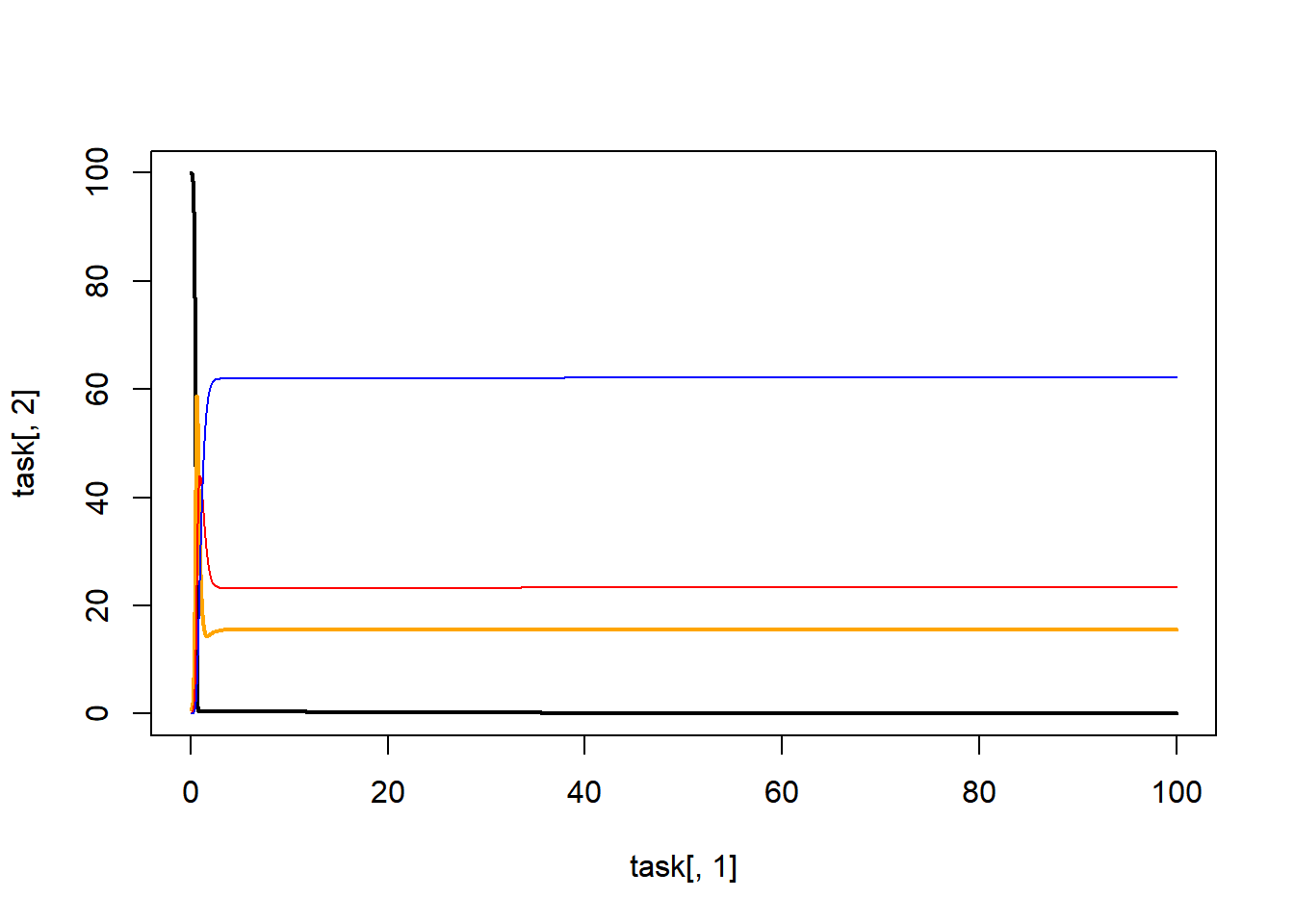#hosts in B
lines(x=task[,1],y=task[,9], col="blue")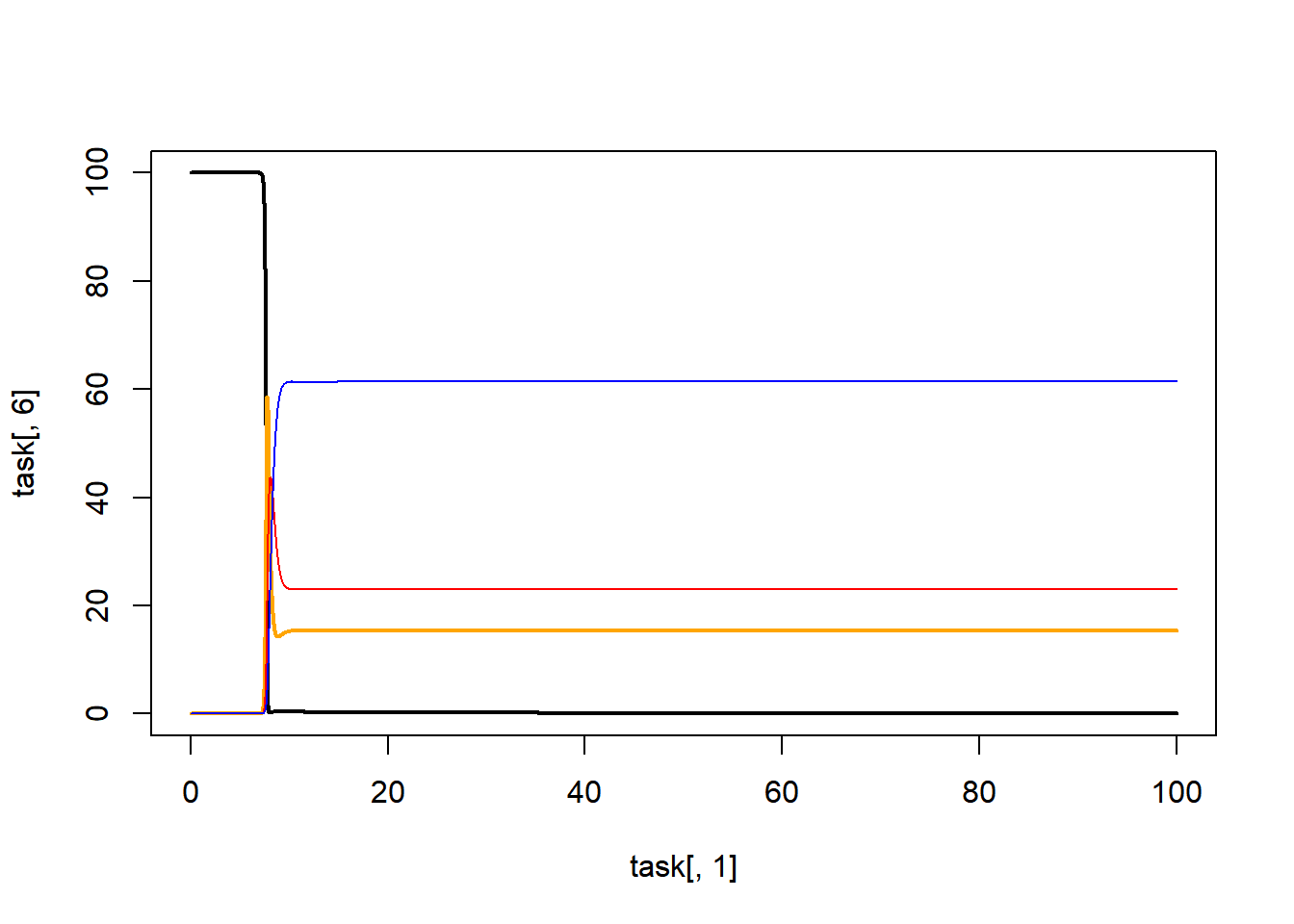#hosts in C
lines(x=task[,1],y=task[,13], col="blue")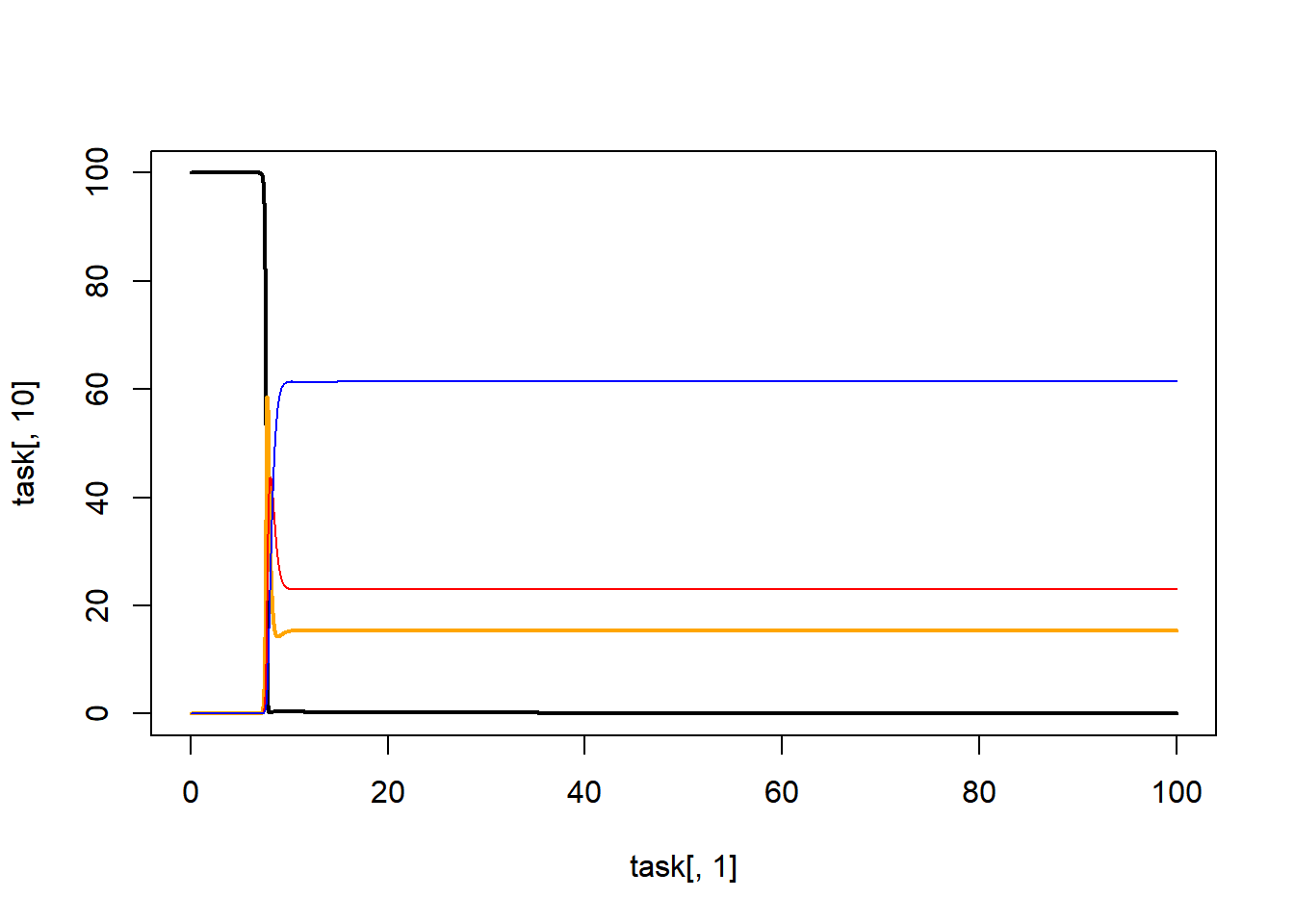#vectors in A
lines(x=task[,1],y=task[,16], col="red")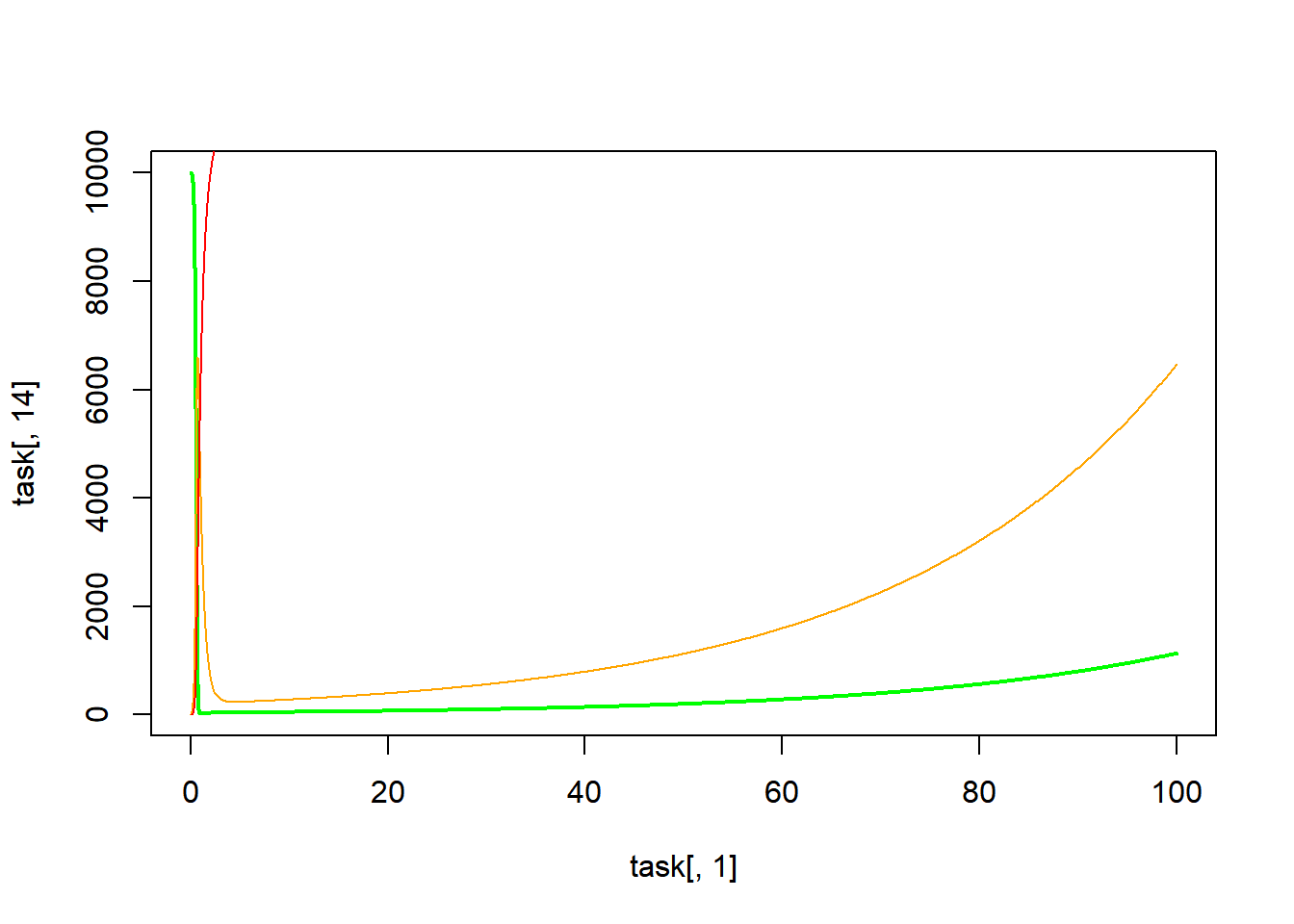#vectors in B
lines(x=task[,1],y=task[,19], col="red")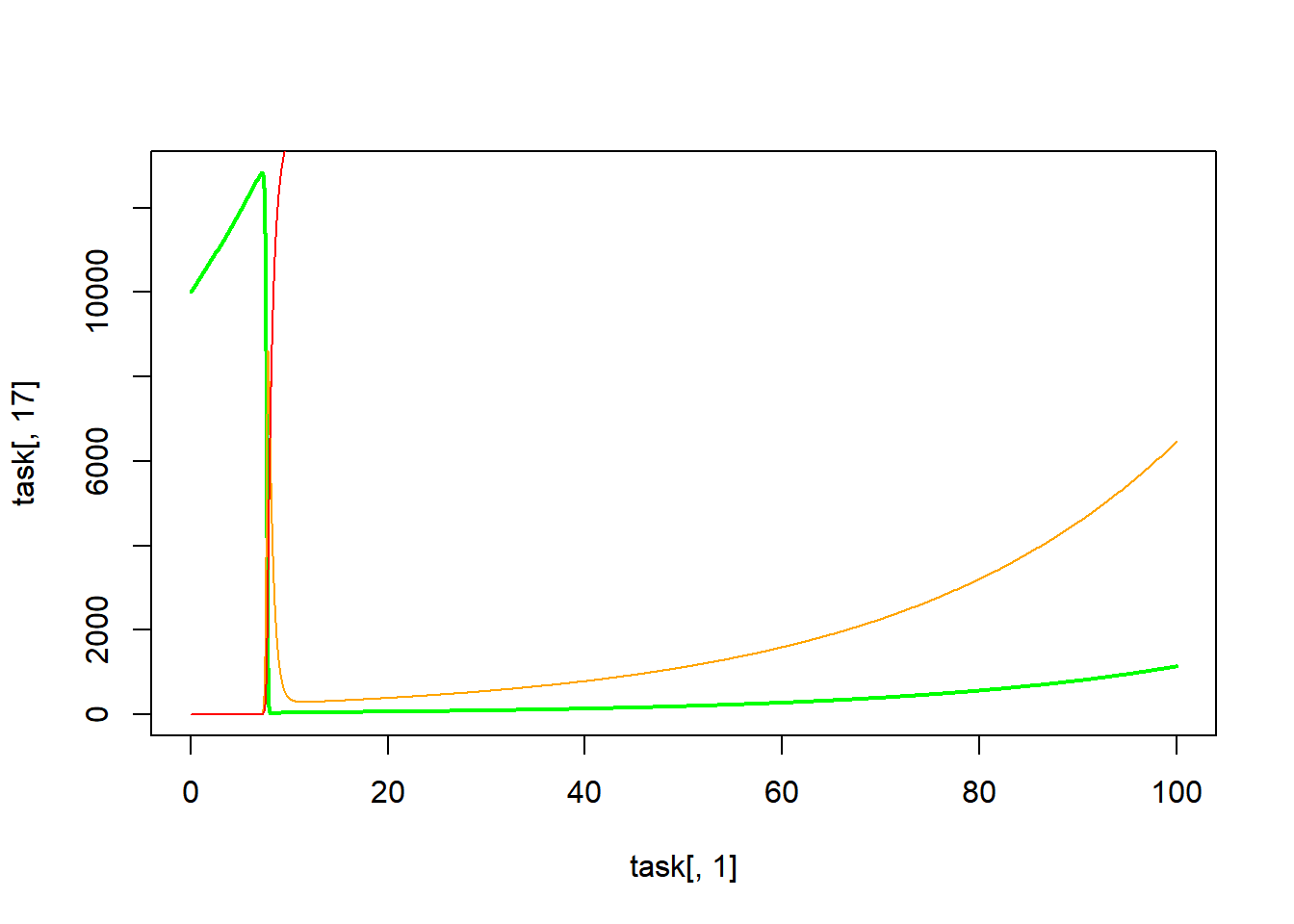#vectors in A
lines(x=task[,1],y=task[,22], col="red")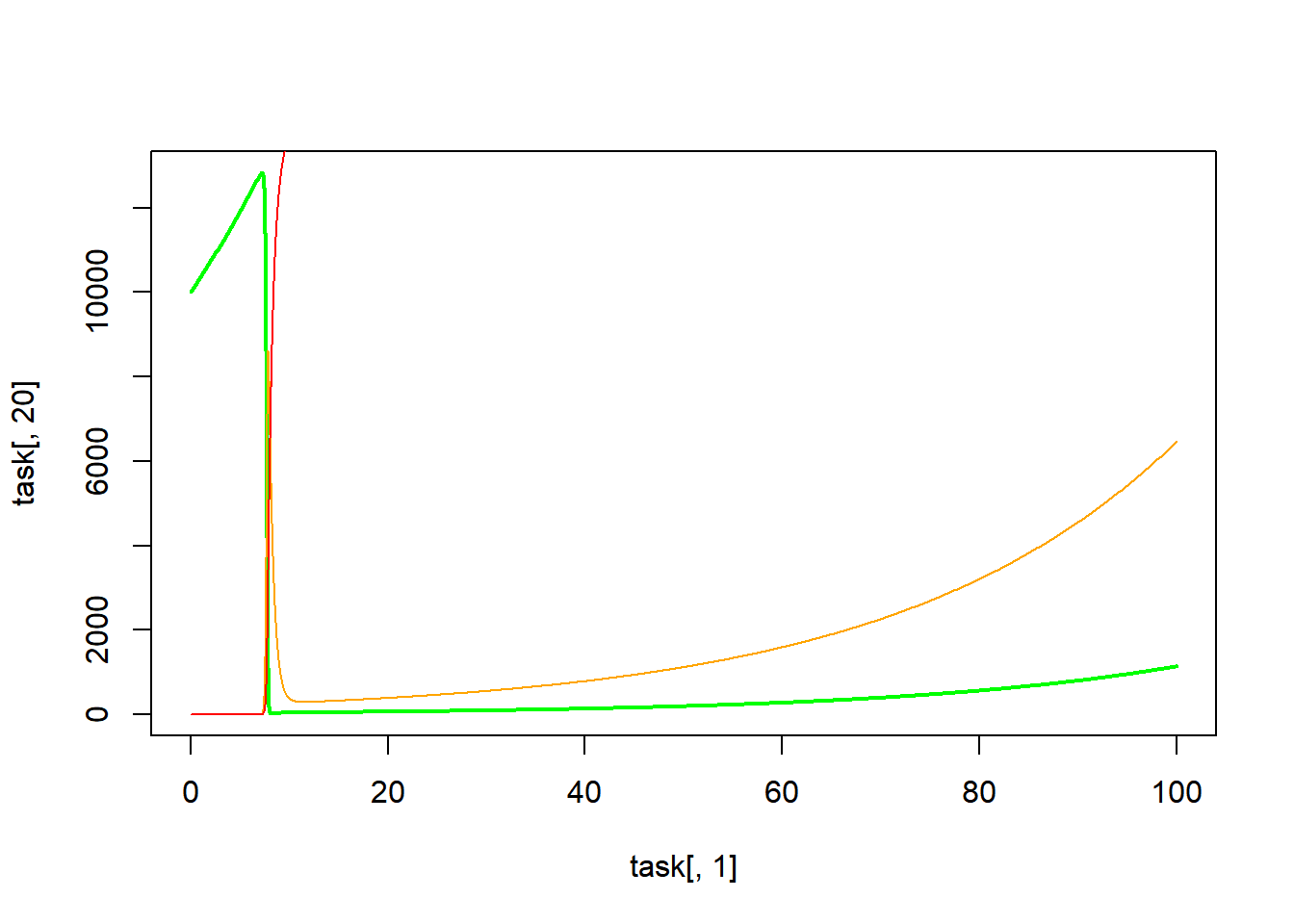1. Manipulate the coupling parameters so that 95% of population A remains in A and the remaining 5% are equally dispersed to B and C.

2. Manipulate the coupling parameters so that 75% of population A remains in A and the remaining 25% are equally dispersed to B and C. How would you describe the differences in 2 and 3? Do they seem more synchronous with increased migration?

3. Set the coupling parameters for domestics equal to 0.34 and all others to 0.33. What do you observe? Is this similar to a simpler model?

4. Play with the coupling parameters so that person migrate from A to B to C, but no other directions.

5. The initial values for this simulation were chosen to reflect malaria transmission. Suppose Population A instituted an intervention for vector control (kill mosquitos). Do efforts of control in Population A affect the overall outbreak(s) downstream? Adjust relevant parameters and find out.

6. Similar to task 6, suppose Population A instituted an intervention for indoor residual spraying. Which parameter might this affect? Are there any downstream effects?

7. Create a composite situation from the scenarios outlined in tasks 6 and 7. Suppose there is an intervention in Population A that halves the vector birth rate and doubles the death rate. Meanwhile, there is an intervention in Populatiuon B that reduces infected host to susceptible vector transmission by a third. Are there any downstream effects?

8. Compare total number of infected persons in Population C from the previous scenarios. Any difference? Synergy?

9. Think of other malaria interventions (such as drug therapy). How would the other interventions affect the parameters in this model? Adjust the model and explore.

### Visitor

Another simulation function was adapted to reflect the “visitor” concept previously mentioned; i.e., native and foreign populations were modeled separately with movement between metapopulations . For sake of simplicity, I transformed the previous simulation to reflect a three-compartment SIR model (hosts) for vector transmission (SI).

A few new parameters were added to account for movement between metapopulations. Unlike the previous model, this simulation does not allow for the instantaneous (?) movement between metapopulations; i.e., movement and transmission are separate processes, rather than movement acting upon both static populations and populations in transit (part of processes) as with the previous simulation. The coupling parameters here represent slightly different concepts than before; there are rate parameters for emigration (exit), immigration (entry), and return to homeland.

Additionally for each movement process of the infected, there is a illness parameter to reflect impact on movement due to infection. Inherently, the model expects the illness parameter to reflect reduction of emigration for infected individuals with an increase in the return rate.

METAPOP.eq2 <- function(t, y, parms)
{
with(
as.list(c(y,parms)),
{
#the ordinary differential equations

dSh.An = - Sh.An * Iv.A * b1.An   +   Rh.An *  w.A   -   em.A * Sh.An                    +   (Sh.Bf + Sh.Cf) * ret
dIh.An =   Sh.An * Iv.A * b1.An   -   Ih.An *  g.A   -   em.A * Ih.An * ill              +   (Ih.Bf + Ih.Cf) * ret * (1 / ill)
dRh.An =   Ih.An *  g.A           -   Rh.An *  w.A   -   em.A * Rh.An                    +   (Rh.Bf + Rh.Cf) * ret

dSh.Af = - Sh.Af * Iv.A * b1.Af   +   Rh.Af *  w.A   +   (Sh.Bn + Sh.Cn) * imm.A         -   Sh.Af * ret
dIh.Af =   Sh.Af * Iv.A * b1.Af   -   Ih.Af *  g.A   +   (Ih.Bn + Ih.Cn) * imm.A * ill   -   Ih.Af * ret * (1 / ill)
dRh.Af =   Ih.Af *  g.A           -   Rh.Af *  w.A   +   (Rh.Bn + Rh.Cn) * imm.A         -   Rh.Af * ret

dSv.A = - Sv.A * b2.A * (Ih.An + Ih.Af)   -   dr.A * Sv.A   +   br.A
dIv.A =   Sv.A * b2.A * (Ih.An + Ih.Af)   -   Iv.A *  dr.A

dSh.Bn = - Sh.Bn * Iv.B * b1.Bn   +   Rh.Bn *  w.B   -   em.B * Sh.Bn                    +   (Sh.Af + Sh.Cf) * ret
dIh.Bn =   Sh.Bn * Iv.B * b1.Bn   -   Ih.Bn *  g.B   -   em.B * Ih.Bn * ill              +   (Ih.Af + Ih.Cf) * ret * (1 / ill)
dRh.Bn =   Ih.Bn *  g.B           -   Rh.Bn *  w.B   -   em.B * Rh.Bn                    +   (Rh.Af + Rh.Cf) * ret

dSh.Bf = - Sh.Bf * Iv.B * b1.Bf   +   Rh.Bf *  w.B   +   (Sh.An + Sh.Cn) * imm.B         -   Sh.Bf * ret
dIh.Bf =   Sh.Bf * Iv.B * b1.Bf   -   Ih.Bf *  g.B   +   (Ih.An + Ih.Cn) * imm.B * ill   -   Ih.Bf * ret * (1 / ill)
dRh.Bf =   Ih.Bf *  g.B           -   Rh.Bf *  w.B   +   (Rh.An + Rh.Cn) * imm.B         -   Rh.Bf * ret

dSv.B = - Sv.B * b2.B * (Ih.Bn + Ih.Bf)   -   dr.B * Sv.B   +   br.B
dIv.B =   Sv.B * b2.B * (Ih.Bn + Ih.Bf)   -   Iv.B *  dr.B

dSh.Cn = - Sh.Cn * Iv.C * b1.Cn   +   Rh.Cn *  w.C   -   em.C * Sh.Cn                    +   (Sh.Af + Sh.Bf) * ret
dIh.Cn =   Sh.Cn * Iv.C * b1.Cn   -   Ih.Cn *  g.C   -   em.C * Ih.Cn * ill              +   (Ih.Af + Ih.Bf) * ret * (1 / ill)
dRh.Cn =   Ih.Cn *  g.C           -   Rh.Cn *  w.C   -   em.C * Rh.Cn                    +   (Rh.Af + Rh.Bf) * ret

dSh.Cf = - Sh.Cf * Iv.C * b1.Cf   +   Rh.Cf *  w.C   +   (Sh.An + Sh.Bn) * imm.C         -   Sh.Cf * ret
dIh.Cf =   Sh.Cf * Iv.C * b1.Cf   -   Ih.Cf *  g.C   +   (Ih.An + Ih.Bn) * imm.C * ill   -   Ih.Cf * ret * (1 / ill)
dRh.Cf =   Ih.Cf *  g.C           -   Rh.Cf *  w.C   +   (Rh.An + Rh.Bn) * imm.C         -   Rh.Cf * ret

dSv.C = - Sv.C * b2.C * (Ih.Cn + Ih.Cf)   -   dr.C * Sv.C   +   br.C
dIv.C =   Sv.C * b2.C * (Ih.Cn + Ih.Cf)   -   Iv.C *  dr.C

list(c(dSh.An, dIh.An, dRh.An,   dSh.Af, dIh.Af, dRh.Af,   dSv.A, dIv.A,
dSh.Bn, dIh.Bn, dRh.Bn,   dSh.Bf, dIh.Bf, dRh.Bf,   dSv.B, dIv.B,
dSh.Cn, dIh.Cn, dRh.Cn,   dSh.Cf, dIh.Cf, dRh.Cf,   dSv.C, dIv.C))
}
)
}

#' Simulation of a compartmental infectious disease transmission model illustrating metapopulation dynamics
#'
#' @description  This model allows for the simulation of a vector-borne infectious disease with metapopulation dynamics
#'
#'
#' @param Sh0.*n initial number of susceptible native hosts in population *
#' @param Ih0.*n initial number of infectious native hosts in population *
#' @param Rh0.*n initial number of recovered native hosts in population *
#'
#' @param Sh0.*f initial number of susceptible foreign hosts in population *
#' @param Ih0.*f initial number of infectious foreign hosts in population *
#' @param Rh0.*f initial number of recovered foreign hosts in population *
#'
#'
#' @param Sv0.* initial number of susceptible vectors in population *
#' @param Iv0.* initial number of infected vectors in population *
#'
#'
#' @param tmax maximum simulation time, units of months
#'
#'
#' @param b1.*n rate of transmission from infected vector to susceptible native host in population *
#' @param b1.*f rate of transmission from infected vector to susceptible foreign host in population *
#' @param b2.* rate of transmission from infected host to susceptible vector in population *
#'
#' @param br.* the rate of births of vectors in population *
#' @param dr.* the rate of death of vectors in population *
#'
#' @param g.* the rate at which infected hosts recover in population *
#' @param w.* the rate at which host immunity wanes in population *
#'
#' @param em.* the rate of emigration (egress) of native hosts from population *
#' @param imm.* the rate of immigration (entry) of foreign hosts to population *
#'
#' @param ill a factor adjusting movement of infected hosts
#' @param ret the rate of return, foreign hosts return to native population
#'
#'
#' @return This function returns the simulation result as obtained from a call
#'   to the deSolve ode solver
#' @details A compartmental ID model with several states/compartments
#'   is simulated as a set of ordinary differential
#'   equations. The compartments are Sh.*, Eh.*, Ih.*, Rh.*, and Sv.*, Ev.*, Iv.* across * populations.
#'   The function returns the output from the odesolver as a matrix,
#'   with one column per compartment/variable. The first column is time.
#' @section Warning:
#'   This function does not perform any error checking. So if you try to do
#'   something nonsensical (e.g. any negative values or fractions > 1),
#'   the code will likely abort with an error message
#' @examples
#'   # To run the simulation with default parameters just call this function
#'   result <- simulate_metapopulation()
#'   # To choose parameter values other than the standard one, specify them e.g. like such
#'   result <- simulate_metapopulation(Sh0.A = 100, Sv0.A = 1e5,  tmax = 100)
#'   # You should then use the simulation result returned from the function, e.g. like this:
#'   plot(result[,1],result[,2],xlab='Time',ylab='Number Susceptible',type='l')
#' @seealso The UI of the shiny app 'Metapopulation', which is part of this package, contains more details on the model
#' @author Cody Dailey, Andreas Handel
#' @references See the information in the corresponding shiny app for model details
#'            See the documentation for the deSolve package for details on ODE solvers
#' @export

simulate_metapopulation_foreign <- function(
Sh0.An = 1e2,      Sh0.Bn = 1e2,      Sh0.Cn = 1e2,
Ih0.An = 0,        Ih0.Bn = 0,        Ih0.Cn = 0,
Rh0.An = 0,        Rh0.Bn = 0,        Rh0.Cn = 0,

Sh0.Af = 1e2,      Sh0.Bf = 1e2,      Sh0.Cf = 1e2,
Ih0.Af = 0,        Ih0.Bf = 0,        Ih0.Cf = 0,
Rh0.Af = 0,        Rh0.Bf = 0,        Rh0.Cf = 0,

Sv0.A = 1e2,       Sv0.B = 1e2,       Sv0.C = 1e2,
Iv0.A = 0,         Iv0.B = 0,         Iv0.C = 0,

tmax = 1000,

b1.An = 0.01,      b1.Bn = 0.01,      b1.Cn = 0.01,
b1.Af = 0.01,      b1.Bf = 0.01,      b1.Cf = 0.01,
b2.A = 0.5,        b2.B = 0.5,        b2.C = 0.5,

br.A = 1/25,       br.B = 1/25,       br.C = 1/25,
dr.A = 0.001,      dr.B = 0.001,      dr.C = 0.001,

g.A = 2,           g.B = 2,           g.C = 2,
w.A = 0,        w.B = 0,        w.C = 0,

em.A = 0 ,         em.B = 0,          em.C = 0,
imm.A = 0 ,        imm.B = 0,         imm.C = 0,

ill = 1,
ret = 0
)
{
############################################################

Y0 = c(Sh.An = Sh0.An, Ih.An = Ih0.An, Rh.An = Rh0.An,
Sh.Af = Sh0.Af, Ih.Af = Ih0.Af, Rh.Af = Rh0.Af,
Sv.A = Sv0.A, Iv.A = Iv0.A,
Sh.Bn = Sh0.Bn, Ih.Bn = Ih0.Bn, Rh.Bn = Rh0.Bn,
Sh.Bf = Sh0.Bf, Ih.Bf = Ih0.Bf, Rh.Bf = Rh0.Bf,
Sv.B = Sv0.B, Iv.B = Iv0.B,
Sh.Cn = Sh0.Cn, Ih.Cn = Ih0.Cn, Rh.Cn = Rh0.Cn,
Sh.Cf = Sh0.Cf, Ih.Cf = Ih0.Cf, Rh.Cf = Rh0.Cf,
Sv.C = Sv0.C, Iv.C = Iv0.C);  #combine initial conditions into a vector

dt = min(0.1, tmax / 1000); #time step for which to get results back

timevec = seq(0, tmax, dt); #vector of times for which solution is returned (not that internal timestep of the integrator is different)

############################################################
#vector of parameters which is sent to the ODE function
pars=c(b1.An = b1.An, b1.Bn = b1.Bn, b1.Cn = b1.Cn,
b1.Af = b1.Af, b1.Bf = b1.Bf, b1.Cf = b1.Cf,
b2.A = b2.A, b2.B = b2.B, b2.C = b2.C,

br.A = br.A, br.B = br.B, br.C = br.C,
dr.A = dr.A, dr.B = dr.B, dr.C = dr.C,

g.A = g.A, g.B = g.B, g.C = g.C,
w.A = w.A, w.B = w.B, w.C = w.C,

ill = ill,
ret = ret,

em.A = em.A, em.B = em.B, em.C = em.C,
imm.A = imm.A, imm.B = imm.B, imm.C = imm.C
);

#this line runs the simulation, i.e. integrates the differential equations describing the infection process
#the result is saved in the odeoutput matrix, with the 1st column the time, the 2nd, 3rd, 4th column the variables S, I, R
#This odeoutput matrix will be re-created every time you run the code, so any previous results will be overwritten
odeoutput = deSolve::lsoda(Y0, timevec, func = METAPOP.eq2, parms=pars, atol=1e-12, rtol=1e-12);

return (odeoutput)
}

1. Adjust the initial settings for steady-state infection rates in population A.
test <- as.data.frame(simulate_metapopulation_foreign(
Sh0.An = 1e2,      Sh0.Bn = 1e2,      Sh0.Cn = 1e2,
Ih0.An = 0,        Ih0.Bn = 0,        Ih0.Cn = 0,
Rh0.An = 0,        Rh0.Bn = 0,        Rh0.Cn = 0,

Sh0.Af = 1e2,      Sh0.Bf = 1e2,      Sh0.Cf = 1e2,
Ih0.Af = 0,        Ih0.Bf = 0,        Ih0.Cf = 0,
Rh0.Af = 0,        Rh0.Bf = 0,        Rh0.Cf = 0,

Sv0.A = 1e2,       Sv0.B = 1e2,       Sv0.C = 1e2,
Iv0.A = 1,         Iv0.B = 0,         Iv0.C = 0,

tmax = 1000,

b1.An = 0.001,      b1.Bn = 0.01,      b1.Cn = 0.01,
b1.Af = 0.001,      b1.Bf = 0.01,      b1.Cf = 0.01,
b2.A = 0.05,        b2.B = 0.5,        b2.C = 0.5,

br.A = 1/25,       br.B = 1/25,       br.C = 1/25,
dr.A = 0.001,      dr.B = 0.001,      dr.C = 0.001,

g.A = 2,           g.B = 2,           g.C = 2,
w.A = 0.75,        w.B = 0.75,        w.C = 0.75,

em.A = 0 ,         em.B = 0,          em.C = 0,
imm.A = 0 ,        imm.B = 0,         imm.C = 0,

ill = 1,
ret = 0))
#popA
plot(x=test[,1], y=test[,2], type="l", ylim=c(0,100)) #sn
lines(x=test[,1], y=test[,5], lty=2)                  #sf
lines(x=test[,1], y=test[,3], lty=1, col="red")       #in
lines(x=test[,1], y=test[,6], lty=2, col="red")       #if
lines(x=test[,1], y=test[,4], lty=1, col="blue")      #rn
lines(x=test[,1], y=test[,7], lty=2, col="blue")      #rf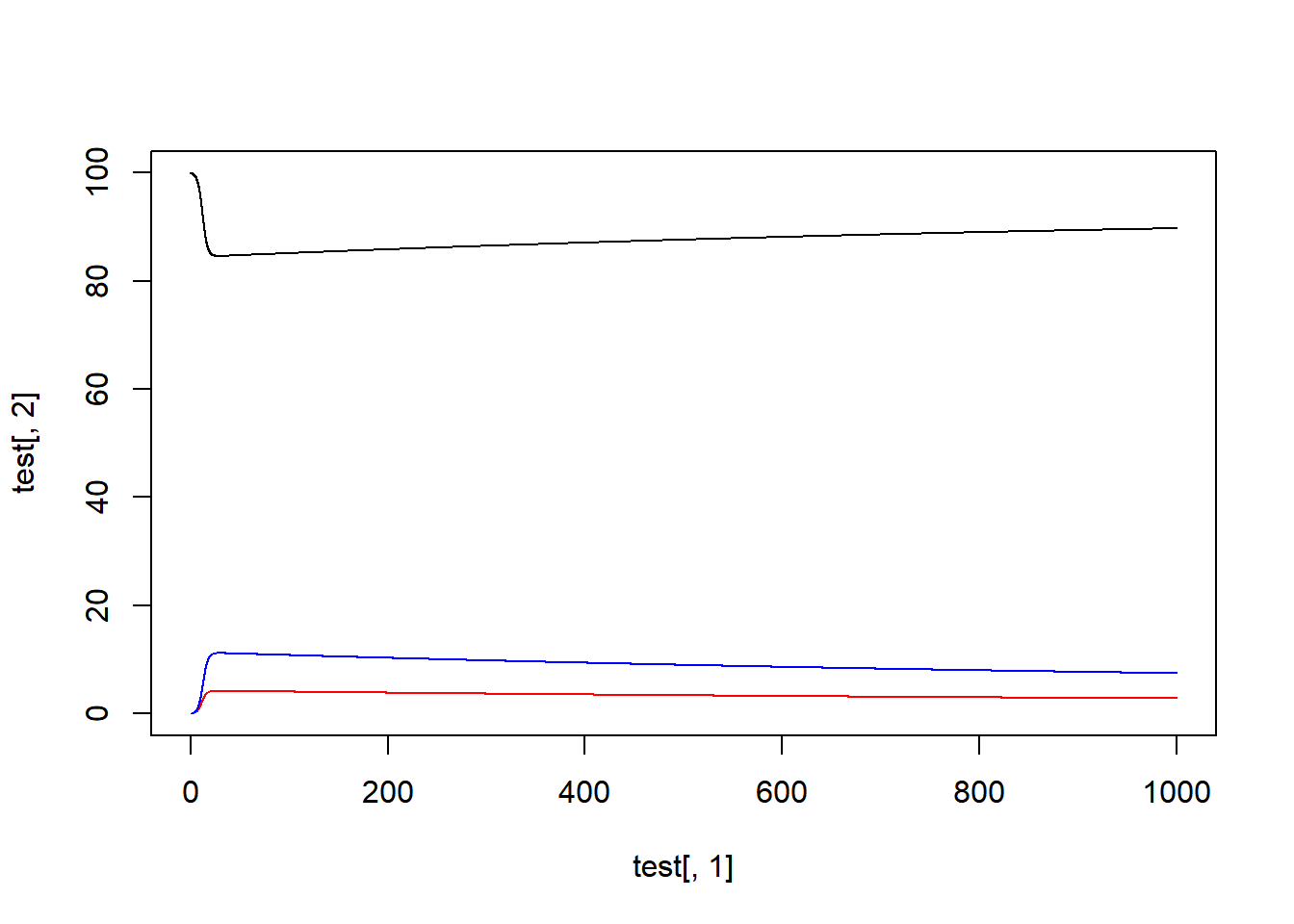1. Adjust the movement parameters to reflect equal movement between A,B,C.

2. Set $$ill=0.05$$. What does this change reflect?

3. Adjust the $$b2.Af$$ parameter to reflect a factor 10 increase in transmission rate for foreigners compared to natives.

4. Play with the parameters so that transmission can only occur in population A. How might endemic disease in population A establish in the other populations?

test <- as.data.frame(simulate_metapopulation_foreign(
Sh0.An = 1e2,      Sh0.Bn = 1e2,      Sh0.Cn = 1e2,
Ih0.An = 0,        Ih0.Bn = 0,        Ih0.Cn = 0,
Rh0.An = 0,        Rh0.Bn = 0,        Rh0.Cn = 0,

Sh0.Af = 1e2,      Sh0.Bf = 1e2,      Sh0.Cf = 1e2,
Ih0.Af = 0,        Ih0.Bf = 0,        Ih0.Cf = 0,
Rh0.Af = 0,        Rh0.Bf = 0,        Rh0.Cf = 0,

Sv0.A = 1e2,       Sv0.B = 1e2,       Sv0.C = 1e2,
Iv0.A = 1,         Iv0.B = 0,         Iv0.C = 0,

tmax = 120,

b1.An = 0.01,      b1.Bn = 0.01,      b1.Cn = 0.01,
b1.Af = 0.01,      b1.Bf = 0.01,      b1.Cf = 0.01,
b2.A = 0.5,        b2.B = 0.5,        b2.C = 0.5,

br.A = 1/25,       br.B = 1/25,       br.C = 1/25,
dr.A = 0.001,      dr.B = 0.001,      dr.C = 0.001,

g.A = 2,           g.B = 2,           g.C = 2,
w.A = 0.75,        w.B = 0.75,        w.C = 0.75,

em.A = 0.0005 ,     em.B = 0.0005,      em.C = 0.0005,
imm.A = 0.001 ,     imm.B = 0.001,      imm.C = 0.001,

ill = 0.5,
ret = 0.001))
#popA
plot(x=test[,1], y=test[,2], type="l", ylim=c(0,150)) #sn
lines(x=test[,1], y=test[,5], lty=2)                  #sf
lines(x=test[,1], y=test[,3], lty=1, col="red")       #in
lines(x=test[,1], y=test[,6], lty=2, col="red")       #if
lines(x=test[,1], y=test[,4], lty=1, col="blue")      #rn
lines(x=test[,1], y=test[,7], lty=2, col="blue")      #rf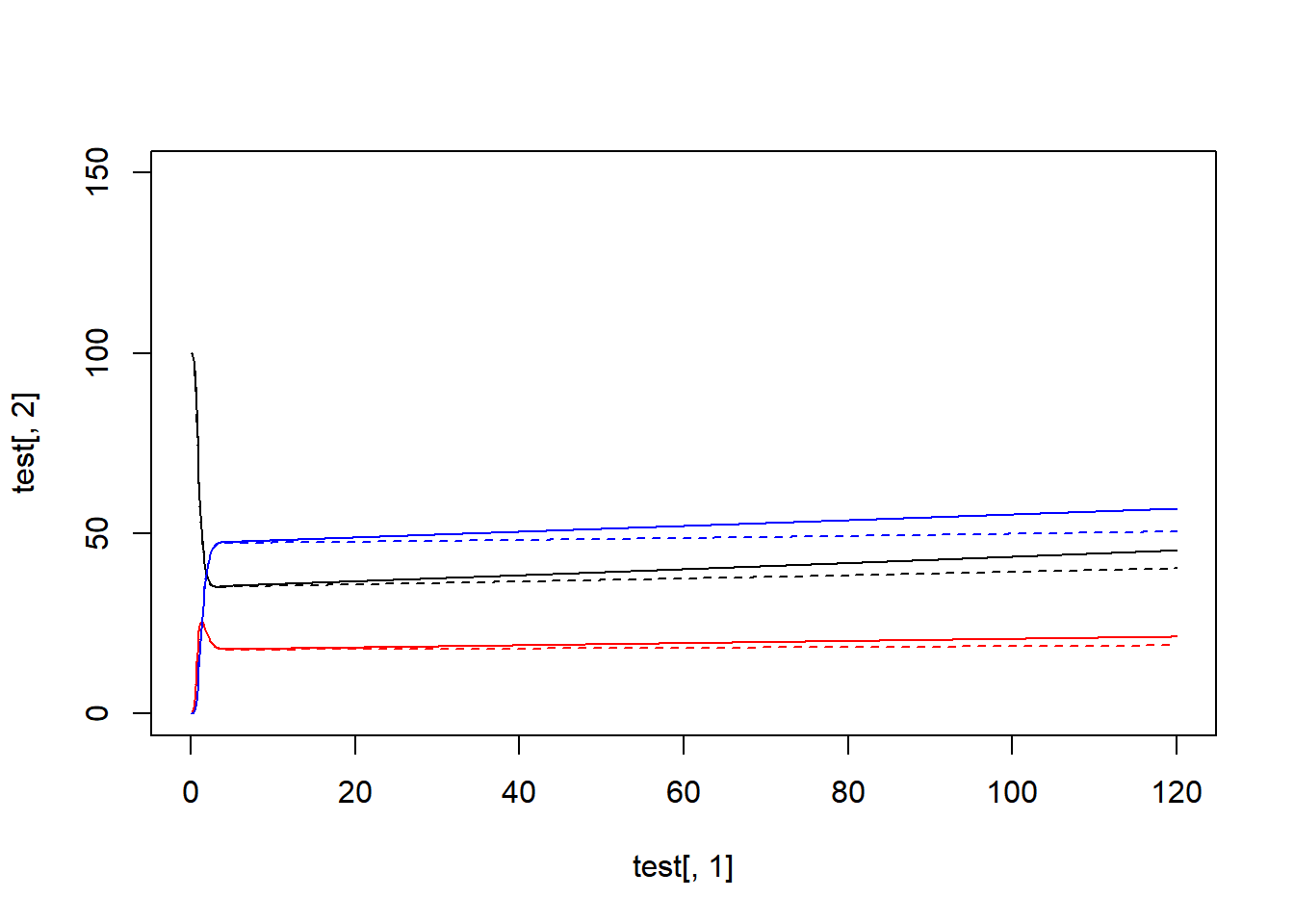#popB
plot(x=test[,1], y=test[,10], type="l", ylim=c(0,150)) #sn
lines(x=test[,1], y=test[,13], lty=2)                  #sf
lines(x=test[,1], y=test[,11], lty=1, col="red")       #in
lines(x=test[,1], y=test[,14], lty=2, col="red")       #if
lines(x=test[,1], y=test[,12], lty=1, col="blue")      #rn
lines(x=test[,1], y=test[,15], lty=2, col="blue")      #rf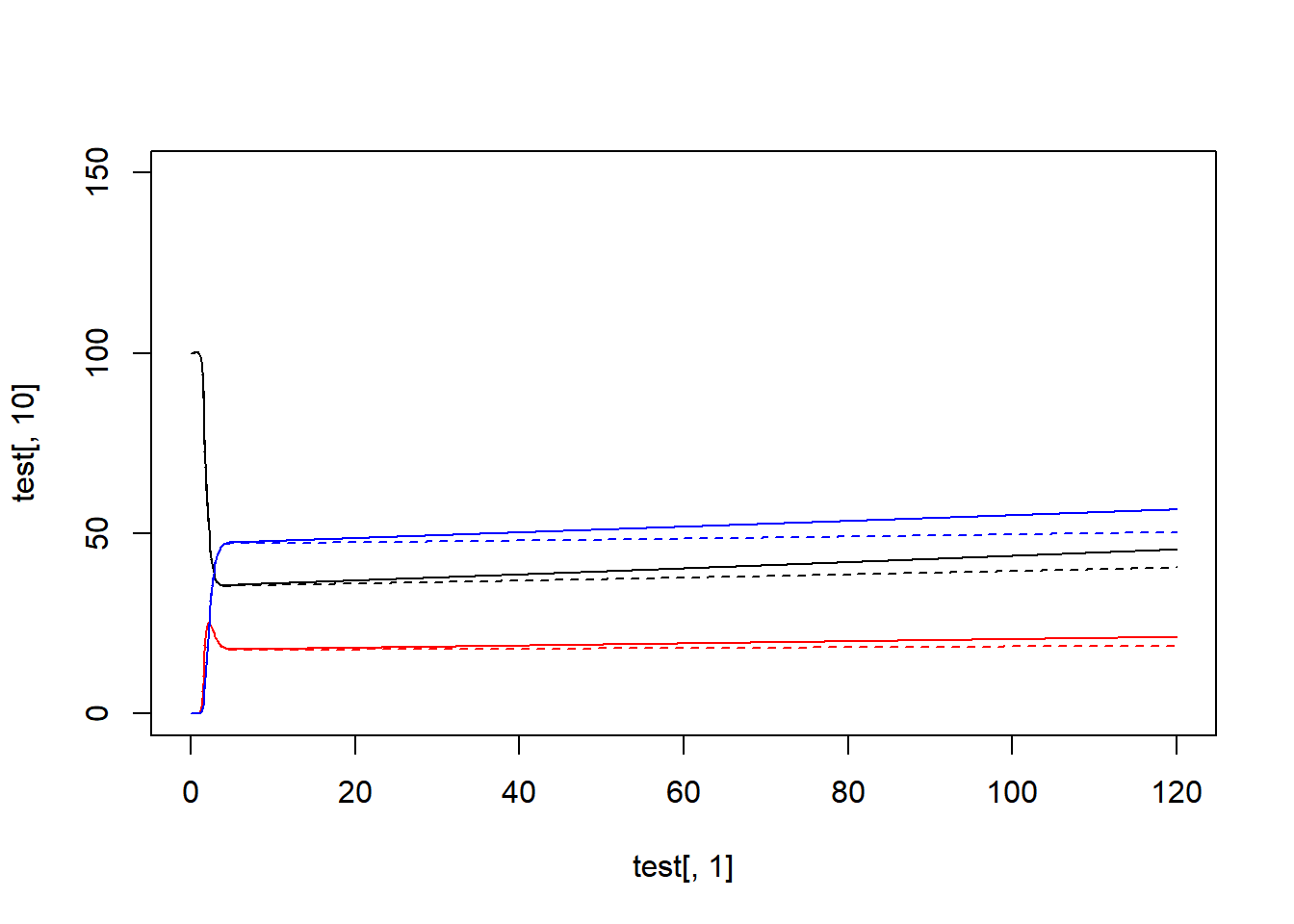#popC
plot(x=test[,1], y=test[,18], type="l", ylim=c(0,150)) #sn
lines(x=test[,1], y=test[,21], lty=2)                  #sf
lines(x=test[,1], y=test[,19], lty=1, col="red")       #in
lines(x=test[,1], y=test[,22], lty=2, col="red")       #if
lines(x=test[,1], y=test[,20], lty=1, col="blue")      #rn
lines(x=test[,1], y=test[,23], lty=2, col="blue")      #rf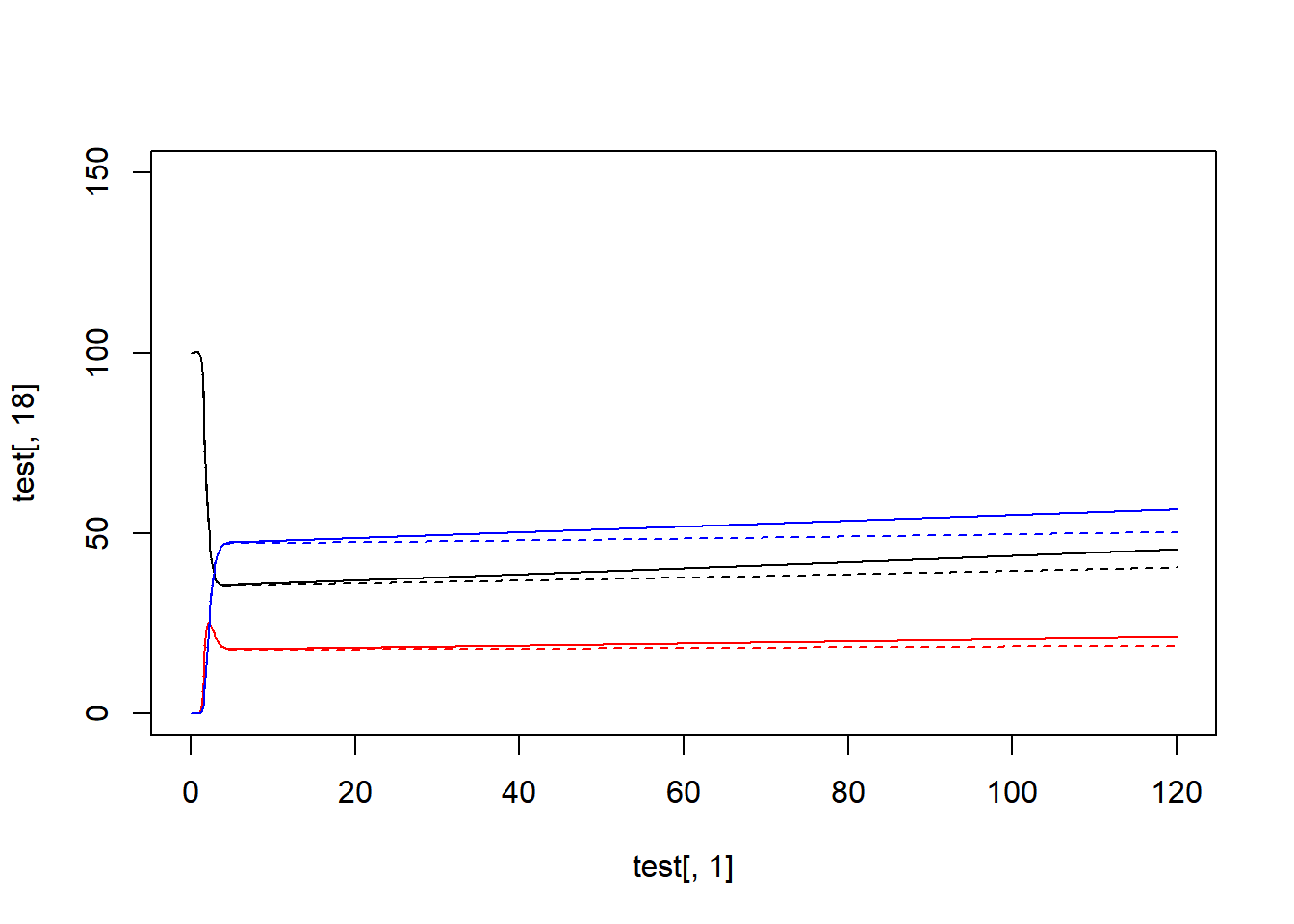These models allow me to explore metapopulation dynamics on vector-borne diseases. The first model for random intermixing could be analogized to an extension of network dynamics to heterogeneous populations. The second model for the “visitor” concept reflects more deeply the heterogeneous populations (natives vs visitors) while accounting for both entry and exit between metapopulations.

Both models reflect a deterministic process by which infinitesmally small changes can exponentiate to greatly affect model conclusion. In this way, this approach offers a limitation. To alleviate this in the future, a stochastic model accounting for the volatility of probability in these concepts will be developed.

## Stepping Stones

• Need to recode so that it’s easier to reflect a finite population.

• Simulation accounting for different types of movement (planes, cars, foot traffic, cruises) and their periodicity (like cruise drops off 500 people at once then they are removed within a few time steps); probably best to use stochastic model.

• Revisit “visitor” concept to model foreigners as more heterogeneous; i.e., the people visiting population A from population B may not demonstrate similar dynamics as someone from population C visiting population A.

• Introduce concept of vector movement.

• Concepts of spatiality and population heterogeneity can complicate the definitions of connectivity. For example, it is common for relatedness to be a quantification of distance which allows for more intimate interaction between close locations than those more distant.